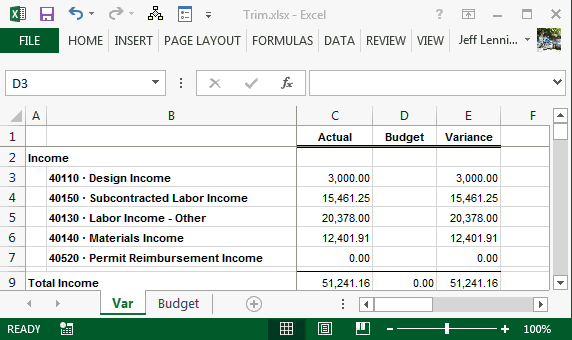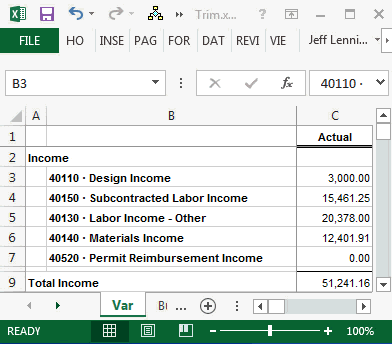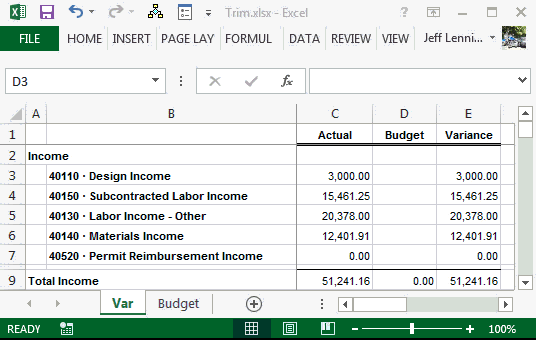# Remove Extra Spaces from Lookup Values with TRIMThis post discusses one way to enable our lookup functions, such as VLOOKUP, to work even when the lookup values contain extra spaces. We’ll use the TRIM function to dynamically remove padding from the lookup values.

## Overview

We’ll use a specific example to demonstrate this function. Let’s say we’ve exported a partial income statement from our accounting system. For some technical reason, it appears that all report labels are padded with trailing spaces so that the total label length is 40 characters. As we inspect each report label, we observe that there are a different number of trailing spaces for each label, as shown below:These extra trailing spaces create problems when we try to perform lookups. Here’s why.

The accounts in the budget table do not have trailing spaces. The budget table (tbl_bud) is shown below:When we write a formula using a lookup function such as VLOOKUP, the lookup value is not found in the lookup range. This is because the lookup value contains the trailing spaces, and the accounts stored in the lookup range do not. To Excel’s lookup functions, these are two different values.

The formulas thus produce errors, as demonstrated below:In order to ensure that the lookup value can be found in the lookup range, we need to remove the trailing spaces. Although we could do this manually, we like to delegate manual tasks to Excel, especially in our recurring use workbooks. So, we enlist the help of the TRIM function. The TRIM function removes any extra spaces, including leading spaces, trailing spaces, and double spaces. The TRIM function has a single argument, the text to trim.

By wrapping a TRIM function around the lookup value, we remove the extra spaces and enable the VLOOKUP function to make its match.

We could use the following formula to perform the lookup:

`=VLOOKUP(TRIM(B3),tbl_bud,2,0)`

Where:

• TRIM(B3) is the lookup value, stripped of extra spaces
• tbl_bud is the lookup range, the budget table
• 2 the column that has the value to return, the amount column
• 0 means exact match

When we use this formula, the budget values are properly returned to our report, as shown below:The TRIM function removes the extra spaces and enables the VLOOKUP function to make its match.

• For other blog posts that reference VLOOKUP
• To handle trailing spaces in the lookup range (instead of the lookup value), check out the wildcard post

Get a quick email notice when a new Excel article is available

• This field is for validation purposes and should be left unchanged.1.Ashley

What if there could be spaces in both the lookup value and the array? Using trim and wildcard together don’t work, for example:

=VLOOKUP(TRIM(A1)&”?”,C:C,1,0)

I’m actually working with an INDEX MATCH function but the same principle applies. TRIM and wildcard functions on their own don’t work either. Also tried adding TRIM and wildcard to the lookup array which just doesn’t work.

Any suggestions?

1.Kurt LeBlanc

Ashley,

I recommend looking into the Power Query capabilities of Excel to handle cleaning the table quickly before writing your formula.

Thanks,
Kurt

2.Vijay

I tried using trim it works perfectly, but the problem is it just edits the spaces in lookup value but not in lookup range. Is there any formula which is used to edit the spaces in lookup range and show the result?

1.Kurt LeBlanc

Hey Vijay,

You can use the Power Query to edit a table and just refresh the Query when you add data! It is an awesome tool in Excel:)

Let me know if you need more help
Kurt LeBlanc

3.Maria

I need help with this one please
Colum D has 2 spaces after the value and when I enter the vlookup formula it gives me n/a
Here’s the formula: =VLOOKUP(AF178,RemHD!D:U,17,FALSE)

1.Kurt LeBlanc

Hey Maria

Excel’s Help Guide suggests that the column in the table you are matching the value to is not the left-most column in the table. That is the major problem with VLOOKUP. My preference is the INDEX/MATCH combo as explained in the blog https://www.excel-university.com/two-dimensional-vlookup/

Also, you will still have an “error” in that the function will not find any matching values for you. The values need to match exactly so the lookup value needs to have the same characters as the table’s values so there’s a couple ways to do this:

First, you can use the Power Query Feature to trim the table’s data in one click. It creates a new table and is ONLY one click more than necessary but will produce the same result as, my personal preference:

In the function, you can join the asterisk (*) to the end of the lookup value to become the lookup value followed by any characters

=VLOOKUP(AF178&”*”,…

Both techniques work, so it is up to you which you like:)

Let me know how that works for you!
Kurt LeBlanc

4.Ines Oswaldo Cruz
5.Anil